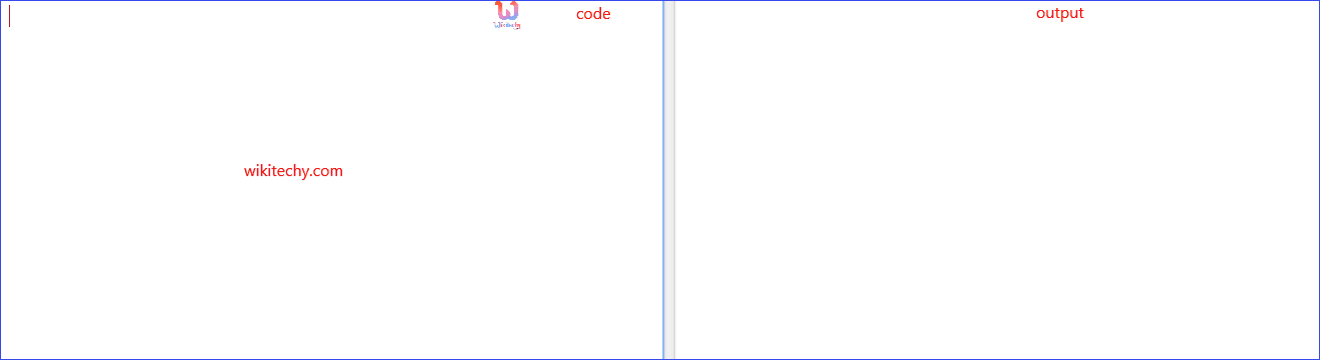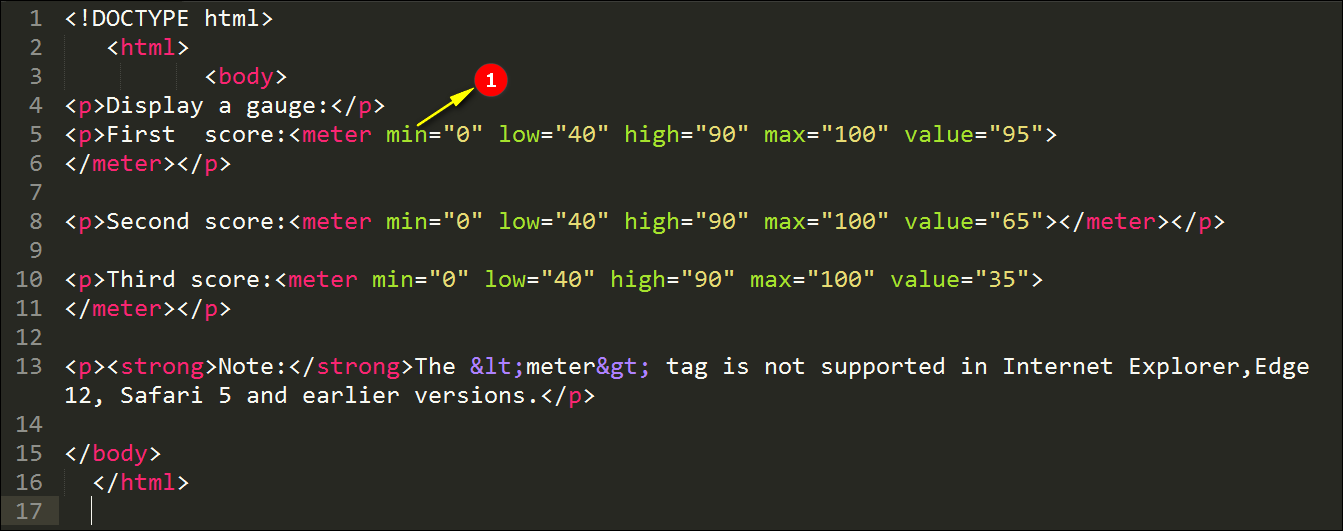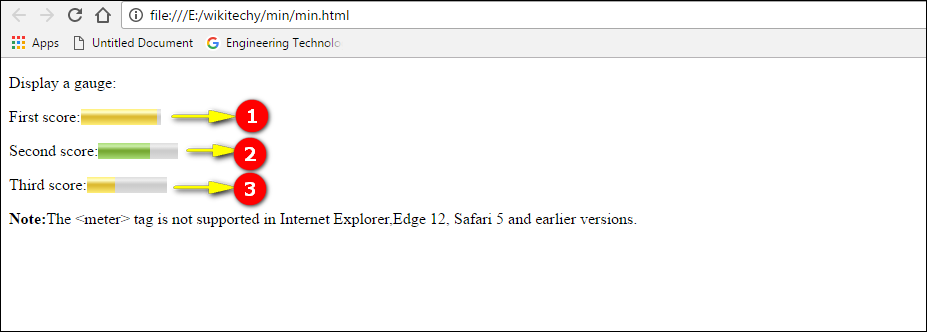# html tutorial - min Attribute in HTML - html5 - html code - html formLearn html - html tutorial - Min attribute in html - html examples - html programs

• The min attribute specifies the minimum value of an element.
• The min attribute when used together with the <meter> element, the min attribute specifies the lower bound of the gauge.
• The min attribute can be used on the following elements. The elements are <input> and <meter>.

## Code Explanation for min attribute in HTML:1. The <min> attribute specifies the minimum value of an element. Here the minimum value given is Zero.

## Sample Output for min attribute in HTML:1. Here the value given for First score is 95, so the shade is maximum in the output as shown above.
2. Here the value given for Second score is 65, so the output displays as above.
3. Here the value given for Third score is 35, so the shade is minimum in the output as shown above.

## Browser Support for autoplay attribute in HTML:4.0 9.0 3.5 4.5 10.5